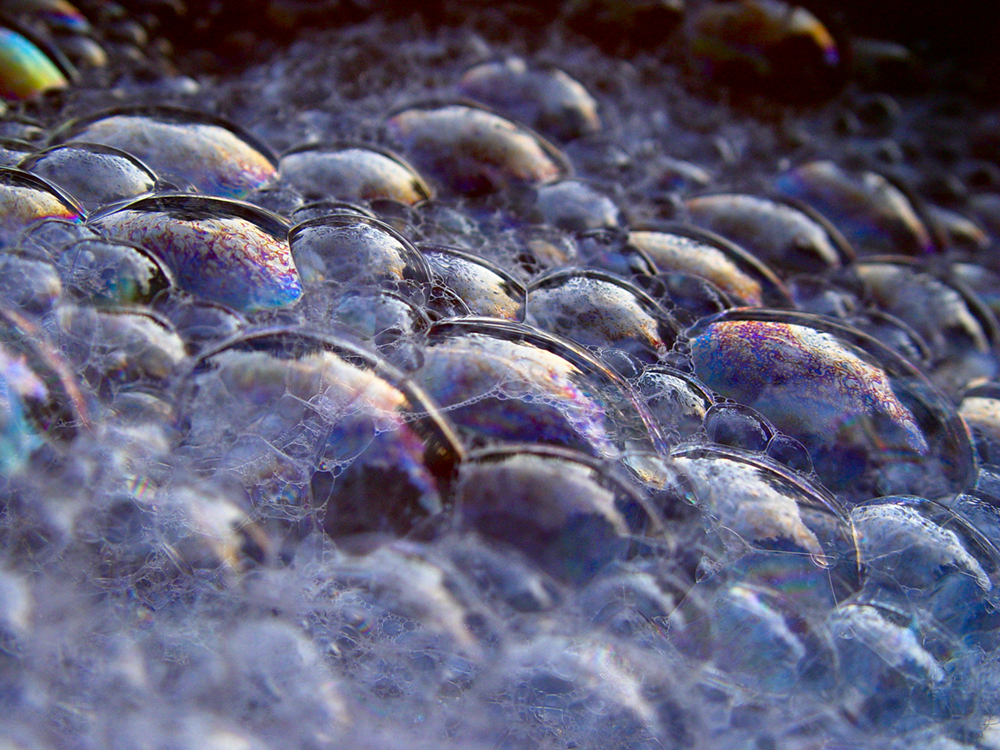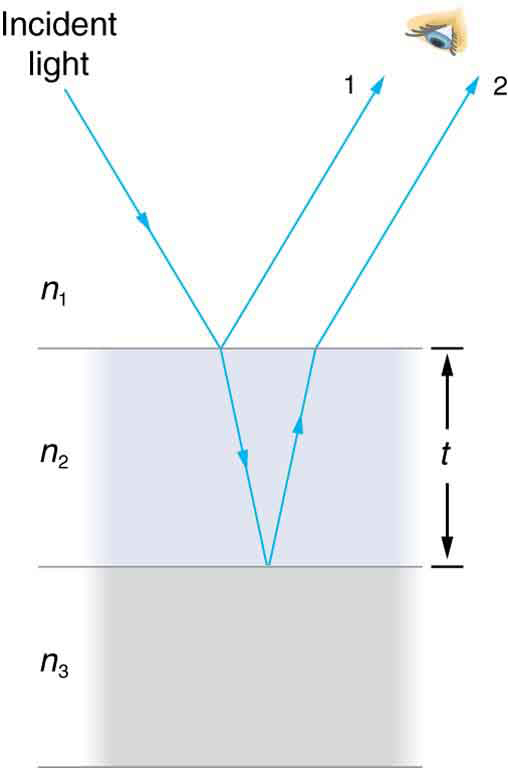# 27.7 Thin film interference

 Page 1 / 6
• Discuss the rainbow formation by thin films.

The bright colors seen in an oil slick floating on water or in a sunlit soap bubble are caused by interference. The brightest colors are those that interfere constructively. This interference is between light reflected from different surfaces of a thin film; thus, the effect is known as thin film interference    . As noticed before, interference effects are most prominent when light interacts with something having a size similar to its wavelength. A thin film is one having a thickness $t$ smaller than a few times the wavelength of light, $\lambda$ . Since color is associated indirectly with $\lambda$ and since all interference depends in some way on the ratio of $\lambda$ to the size of the object involved, we should expect to see different colors for different thicknesses of a film, as in [link] .These soap bubbles exhibit brilliant colors when exposed to sunlight. (credit: Scott Robinson, Flickr)

What causes thin film interference? [link] shows how light reflected from the top and bottom surfaces of a film can interfere. Incident light is only partially reflected from the top surface of the film (ray 1). The remainder enters the film and is itself partially reflected from the bottom surface. Part of the light reflected from the bottom surface can emerge from the top of the film (ray 2) and interfere with light reflected from the top (ray 1). Since the ray that enters the film travels a greater distance, it may be in or out of phase with the ray reflected from the top. However, consider for a moment, again, the bubbles in [link] . The bubbles are darkest where they are thinnest. Furthermore, if you observe a soap bubble carefully, you will note it gets dark at the point where it breaks. For very thin films, the difference in path lengths of ray 1 and ray 2 in [link] is negligible; so why should they interfere destructively and not constructively? The answer is that a phase change can occur upon reflection. The rule is as follows:

When light reflects from a medium having an index of refraction greater than that of the medium in which it is traveling, a $\text{180º}$ phase change (or a $\lambda /2$ shift) occurs.Light striking a thin film is partially reflected (ray 1) and partially refracted at the top surface. The refracted ray is partially reflected at the bottom surface and emerges as ray 2. These rays will interfere in a way that depends on the thickness of the film and the indices of refraction of the various media.

If the film in [link] is a soap bubble (essentially water with air on both sides), then there is a $\lambda /2$ shift for ray 1 and none for ray 2. Thus, when the film is very thin, the path length difference between the two rays is negligible, they are exactly out of phase, and destructive interference will occur at all wavelengths and so the soap bubble will be dark here.

The thickness of the film relative to the wavelength of light is the other crucial factor in thin film interference. Ray 2 in [link] travels a greater distance than ray 1. For light incident perpendicular to the surface, ray 2 travels a distance approximately $2t$ farther than ray 1. When this distance is an integral or half-integral multiple of the wavelength in the medium ( ${\lambda }_{n}=\lambda /n$ , where $\lambda$ is the wavelength in vacuum and $n$ is the index of refraction), constructive or destructive interference occurs, depending also on whether there is a phase change in either ray.

kinetic functional force
what is a principal wave?
A wave the movement of particles on rest position transferring energy from one place to another
Gabche
not wave. i need to know principal wave or waves.
Haider
principle wave is a superposition of wave when two or more waves meet at a point , whose amplitude is the algebraic sum of the amplitude of the waves
kindly define principal wave not principle wave (principle of super position) if u can understand my question
Haider
what is a model?
hi
Muhanned
why are electros emitted only when the frequency of the incident radiation is greater than a certain value
b/c u have to know that for emission of electron need specific amount of energy which are gain by electron for emission . if incident rays have that amount of energy electron can be emitted, otherwise no way.
Nazir
Nazir
what is ohm's law
states that electric current in a given metallic conductor is directly proportional to the potential difference applied between its end, provided that the temperature of the conductor and other physical factors such as length and cross-sectional area remains constant. mathematically V=IR
ANIEFIOK
hi
Gundala
A body travelling at a velocity of 30ms^-1 in a straight line is brought to rest by application of brakes. if it covers a distance of 100m during this period, find the retardation.
just use v^2-u^2=2as
Gundala
how often does electrolyte emits?
alhassan
just use +€^3.7°√π%-4¢•∆¥%
v^2-u^2=2as v=0,u=30,s=100 -30^2=2a*100 -900=200a a=-900/200 a=-4.5m/s^2
akinyemi
what's acceleration
The change in position of an object with respect to time
Mfizi
Acceleration is velocity all over time
Pamilerin
hi
Stephen
It's not It's the change of velocity relative to time
Laura
Velocity is the change of position relative to time
Laura
acceleration it is the rate of change in velocity with time
Stephen
acceleration is change in velocity per rate of time
Noara
what is ohm's law
Stephen
Ohm's law is related to resistance by which volatge is the multiplication of current and resistance ( U=RI)
Laura
acceleration is the rate of change. of displacement with time.
the rate of change of velocity is called acceleration
Asma
how i don understand
how do I access the Multiple Choice Questions? the button never works and the essay one doesn't either
How do you determine the magnitude of force
mass × acceleration OR Work done ÷ distance
Seema
Which eye defect is corrected by a lens having different curvatures in two perpendicular directions?
acute astigmatism?
the difference between virtual work and virtual displacement
How do you calculate uncertainties
What is Elasticity
the property of a body to regain it's original shape is called elasticity. or. the property of a body which can be stretch is called elasticity.
NazirByBy David CoreyBy OpenStaxBy OpenStaxBy Sheila LopezBy P. Wynn NormanBy John GabrieliBy Vanessa SoledadBy Anonymous UserBy Jams KaloBy Carly Allen